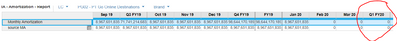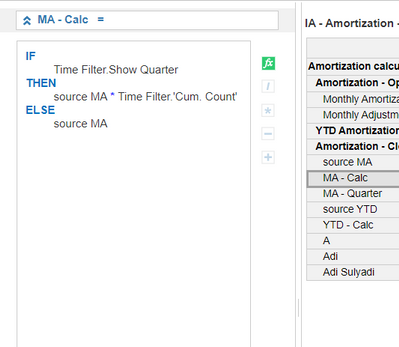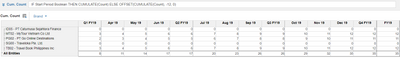# Formula for SummaryI have a case, i want Monthly Amortization Line item can show Quater amount. but not show because on Summary have Sum,Time:Formula. Can give me a answer. Thank you. Help me

Tagged:

•Hi,

I think maybe in your source module you using closing balance, so it will make you Q1 amount will 0.

you can change closing balance to another format.

Thanks

•This would suggest that the source for the 2020 data does not have quarterly totals enabled (no time sum). When using the summary method of Formula, the target module does not calculate the sum but rather references another module/line item for that value.
•So do you have any idea ? I am very confused about that.
•If the formula references another module, then you will need to ensure that the quarterly totals are reflecting there correctly.  Can share the formula on the line item in question?  It's difficult to determine the root issue just looking at the values alone.

•this formula for final report: * time filter. **bleep** count for calculation Quarter, Q1: 3, Q2: 6, Q:9this formula for **bleep**. count•Hi,
I think the best approach is to spread this out over multiple line items.
The Quarterly summary is cumulative while the months are not and this is causing you the issues.
Create the following line items -
1. Monthly
2. Monthly Cumulate

3. Cumulate reset
4. Ref

1. Monthly will house you monthly movement
2. Monthly cumulate will be the cumulative of the 1.Monthly, set summary to SUM, formula = CUMULATE( 1.Monthly, 3. Cumulate reset )
3. Cumulate reset, boolean format and time set to Quarters, formula = true
4. Will contain a formula = 1, set summary to formula

In line item 1. Monthly set your summary to ratio - 2. Monthly Cumulate / 3. Ref
This will result in the your months showing the monthly movement and the quarters to cumulative for that quarter.